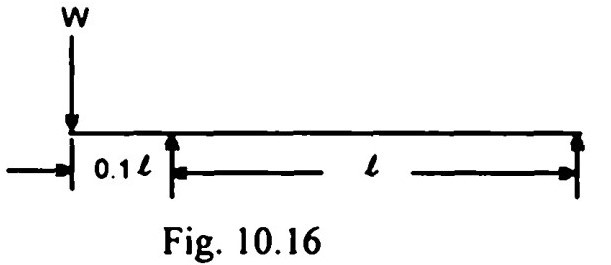Search

# Strength of Materials(141-160)

Updated: Apr 28, 2020

141. For the design of a cast iron member, the most appropriate theory of failure is

Rankine's theory

142. In a uni-dimensional stress system, the principal plane is defined as one on which the

1. shear stress is zero '

2. normal stress is maximum

143.If an element is subjected to pure shearing stress τₓᵧ the maximum principal stress is equal to τₓᵧ

144.A steel cable of 2 cm diameter is used to lift load of 500 n kg. Given that, E = 2 x 106 kg/cm² and the length of the cable is 10 m, the elongation of the cable due to the load will be 0.25 cm

145.A mild steel bar is in three parts, each 20 cm long. The diameters of parts AB, BC and CD are 2 cm, 1 cm and 3 cm respectively. The bar is subjected to an

axial pull of 4t as shown in the Fig.If E =2 x 106 kg/cm² and the elongations in the three parts of the bar are Δ₁, Δ₂ and Δ₃ respectively, then the ratio of the greatest to the least of these elongations

will be 9

146. A steel bar of 2 m length is fixed at both ends at 20°C. The coefficient of thermal expansion is 11 x 10⁻⁶/°C and the modulus of elasticity is 2 x 106 kg/cm². If the temperature is changed to 18°C, then the bar will experience a stress of

44 kg/cm²

147. A cast iron block of 5 sq. cm. cross section carries an axial tensile load of 101.

Then maximum shear stress in the block is given by 1000 kg/cm²

148.An elastic bar of length T, cross sectional area A, Young's modulus of elasticity E and self weight W is hanging vertically. It is subjected to a load P applied axially at the bottom end. The total elongation of the bar is given by

(WI/2AE)+(PI/AE)

149.A long construction member of uniform section is to be lifted using ropes at C and D (see Fig. (a)). This causes bending moment due to self weight as shown in Fig. (b). To minimise the peak value of bending moment, the overhang 'b' shall be such, that M=M

150. Consider the following statements with reference to a continuous beam supported at A, C and E for which the shear force diagram is shown in Fig.1. a concentrated load acting at point B.

2. a uniformly distributed load acting on the portion CE.

151.152. The bending moment diagram for an overhanging beam is shown in Fig.The point of contraflexure would include C and D

153.A Mohr's circle reduces to a point when the body is subjected to uniaxial stress only

154.Fig. 10.16 shows a beam of supported length T and overhang 0.1 I, carrying a

concentrated load W at the end of the overhang. Which one of the following

figures would represent the correct shear force diagram for the beam ?155. A T-section is used as a simply supported beam with uniform loading. The maximum bending stresses for a given load will occur at the bottom of the section

156. An I-section beam, made of structural steel has an overall depth of 30 cm. If the developed flange stresses at the top and bottom of the beam are 1200 kg/cm² and 300 kg/cm² respectively, then the depth of the neutral axis from the top of the beam would be 24 cm

157. Consider the following statements:

A: For beams, I-sections are more economical than rectangular sections.

R : Most of the material is concentrated away from the centroid.

Of these statements

both A and R are true and R is the correct explanation of A

158. The shear stress distribution over a rectangular cross section of a beam follows a parabolic path

159. A simply supported beam of span 'L' and uniform flexural rigidity EI, carries a

central load 'W and total uniformly distributed load 'W throughout the span.

The maximum deflection is given by 13 WL³/384 EI

160. An overhanging beam of overall length L and uniform flexural rigidity EI is loaded by two concentrated loads at the two ends. The beam is supported symmetrically on two supports with overhang 'a' on each side as shown in Fig.Pa (L - 2a)² / 8 EI